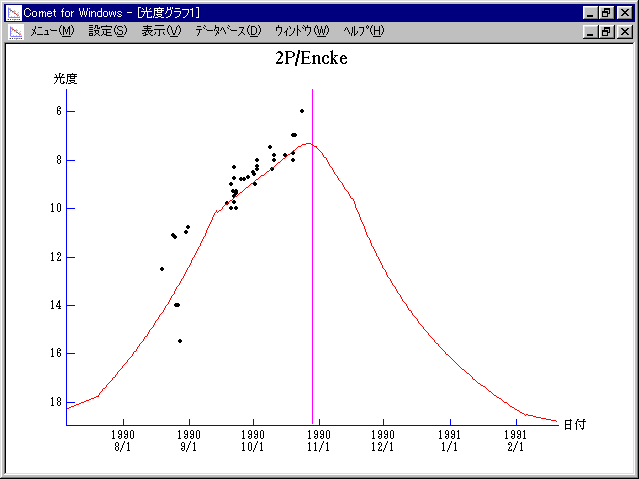# 2P/Encke (1990)###Magnitudes Graph

```        m1 = 15.0 + 5 log d +  5.0 log r  [    ,-100]  (             - 1990 July 20)
m1 =  9.8 + 5 log d + 25   log r  [-100, -45]  (1990 July 20 - 1990 Sept.13)
m1 = 10.3 + 5 log d +  7.0 log r  [ -45,  20]  (1990 Sept.13 - 1990 Nov. 17)
m1 = 12.3 + 5 log d + 15.7 log r  [  20, 100]  (1990 Nov. 17 - 1991 Feb.  5)
m1 = 15.0 + 5 log d +  5.0 log r  [ 100,    ]  (1991 Feb.  5 -             )
```##### The magnitudes graphs are made with Comet for Windows.